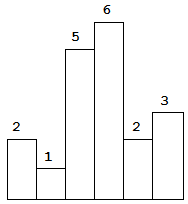# 84 Largest Rectangle in Histogram

## 题目

Given n non-negative integers representing the histogram's bar height where the width of each bar is 1, find the area of largest rectangle in the histogram.Above is a histogram where width of each bar is 1, given height = [2,1,5,6,2,3].The largest rectangle is shown in the shaded area, which has area = 10 unit.

Example:

## 解题思路

(right - left - 1) * height = (6 - 0 - 1) * 2= 8

(遍历一遍 heights 为 n, 每个 heights[i] 还需要分别往左右两侧各跑一次合计为 n.)

(只需要跑一趟)

(6 - (-1) - 1) * 2 = 12

• i 一定是 stack[top] 的 right(右边界, stack[top] 右侧的第一个比 stack[top] 小的值).
• stack[top - 1] 一定是 stack[top] 的 left(左边界, 比 heights[stack[top]] 大的都被弹出去了).

• stack 为获取栈顶元素
• stack 为栈顶的下一个元素
• stack[stack.length - 1] 为获取栈底元素
• stack.unshift() 入栈
• stack.shift() 出栈(获取值并将其从栈顶删除)